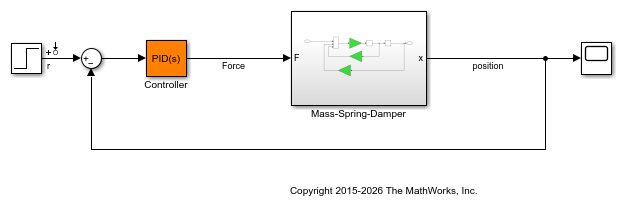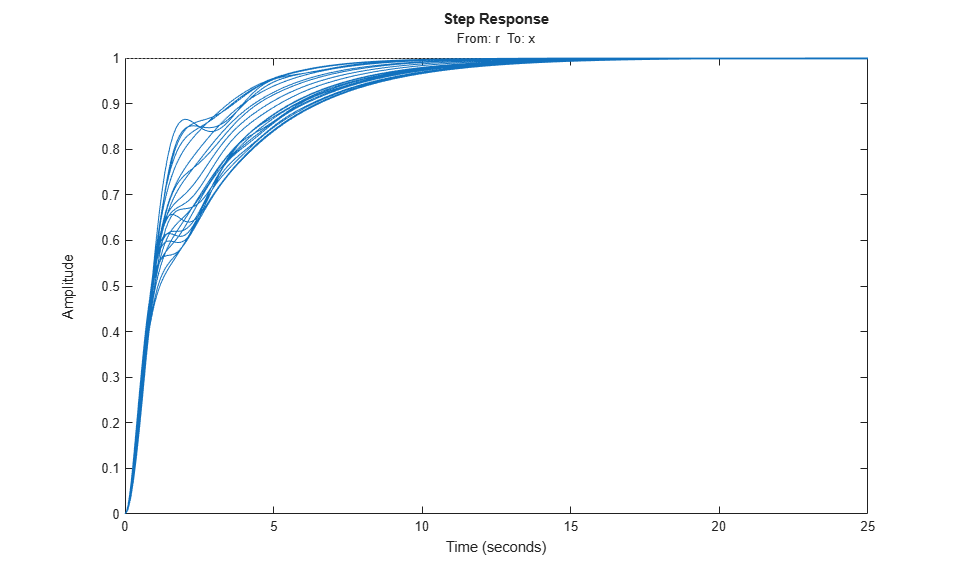Main Content

# Linearize Simulink Block to Uncertain Model

This example shows how to make a Simulink® block linearize to an uncertain variable at the command line. To learn how to specify an uncertain block linearization using the Simulink model editor, see Specify Uncertain Linearization for Core or Custom Simulink Blocks.

For this example, open the Simulink model `slexAircraftExample`.

```mdl = 'slexAircraftExample'; open_system(mdl) ```Examine the subsystem `Aircraft Dynamics Model`.

```subsys = [mdl,'/Aircraft Dynamics Model']; open_system(subsys) ```Suppose you want to specify the following uncertain real values for the gain blocks `Mw` and `Zd`.

```Mw_unc = ureal('Mw',-0.00592,'Percentage',50); Zd_unc = ureal('Zd',-63.9979,'Percentage',30); ```

To specify these values as the linearization for these blocks, create a `BlockSubs` structure to pass to the `linearize` function. The field names are the names of the Simulink blocks, and the values are the corresponding uncertain values. Note that in this model, the name of the `Mw` block is `Gain4`, and the name of the `Zd` block is `Gain5`.

```Mw_name = [subsys,'/Gain4']; Zd_name = [subsys,'/Gain5']; BlockSubs(1).Name = Mw_name; BlockSubs(1).Value = Mw_unc; BlockSubs(2).Name = Zd_name; BlockSubs(2).Value = Zd_unc; ```

Compute the uncertain linearization. `linearize` linearizes the model at operating point specified in the model, making the substitutions specified by `BlockSubs`. The result is an uncertain state-space model with an uncertain real parameter for each of the two uncertain gains.

```sys = linearize(mdl,BlockSubs) ```
```sys = Uncertain continuous-time state-space model with 1 outputs, 1 inputs, 7 states. The model uncertainty consists of the following blocks: Mw: Uncertain real, nominal = -0.00592, variability = [-50,50]%, 1 occurrences Zd: Uncertain real, nominal = -64, variability = [-30,30]%, 1 occurrences Type "sys.NominalValue" to see the nominal value, "get(sys)" to see all properties, and "sys.Uncertainty" to interact with the uncertain elements. ```Examine the uncertain model response.

```step(sys) ````step` takes random samples and provides a sense of the range of responses within the uncertainty of the linearized model.

## See Also

(Simulink Control Design)

## SupportGet trial now